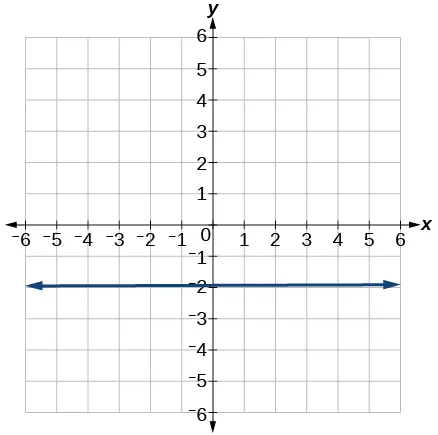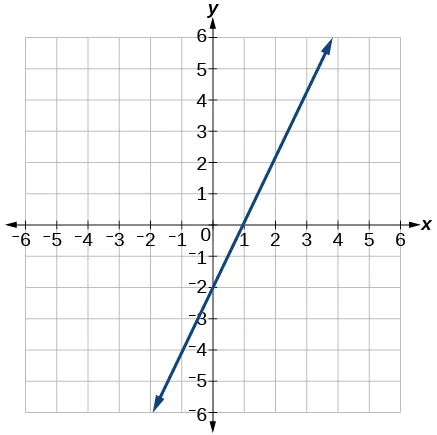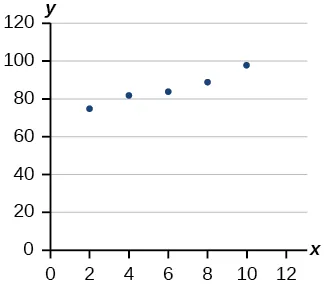Algebra and Trigonometry

# Review Exercises

Algebra and TrigonometryReview Exercises

### Review Exercises

##### Linear Functions
1.

Determine whether the algebraic equation is linear. $2x+3y=7 2x+3y=7$

2.

Determine whether the algebraic equation is linear. $6 x 2 −y=5 6 x 2 −y=5$

3.

Determine whether the function is increasing or decreasing.

$f( x )=7x−2 f( x )=7x−2$

4.

Determine whether the function is increasing or decreasing.

$g( x )=−x+2 g( x )=−x+2$

5.

Given each set of information, find a linear equation that satisfies the given conditions, if possible.

Passes through $( 7,5 ) ( 7,5 )$ and $( 3,17 ) ( 3,17 )$

6.

Given each set of information, find a linear equation that satisfies the given conditions, if possible.

x-intercept at $( 6,0 ) ( 6,0 )$ and y-intercept at $( 0,10 ) ( 0,10 )$

7.

Find the slope of the line shown in the graph.8.

Find the slope of the line graphed.9.

Write an equation in slope-intercept form for the line shown.10.

Does the following table represent a linear function? If so, find the linear equation that models the data.

 x –4 0 2 10 g(x) 18 –2 –12 –52
11.

Does the following table represent a linear function? If so, find the linear equation that models the data.

 x 6 8 12 26 g(x) –8 –12 –18 –46
12.

On June 1st, a company has \$4,000,000 profit. If the company then loses 150,000 dollars per day thereafter in the month of June, what is the company’s profit nth day after June 1st?

For the following exercises, determine whether the lines given by the equations below are parallel, perpendicular, or neither parallel nor perpendicular:

13.

$2x−6y=12 −x+3y=1 2x−6y=12 −x+3y=1$

14.

$y= 1 3 x−2 3x+y=−9 y= 1 3 x−2 3x+y=−9$

For the following exercises, find the x- and y- intercepts of the given equation

15.

$7x+9y=−63 7x+9y=−63$

16.

$f(x)=2x−1 f(x)=2x−1$

For the following exercises, use the descriptions of the pairs of lines to find the slopes of Line 1 and Line 2. Is each pair of lines parallel, perpendicular, or neither?

17.

Line 1: Passes through $(5,11) (5,11)$ and $(10,1) (10,1)$

Line 2: Passes through $(−1,3) (−1,3)$ and $(−5,11) (−5,11)$

18.

Line 1: Passes through $(8,−10) (8,−10)$ and $(0,−26) (0,−26)$

Line 2: Passes through $(2,5) (2,5)$ and $(4,4) (4,4)$

19.

Write an equation for a line perpendicular to $f(x)=5x−1 f(x)=5x−1$ and passing through the point (5, 20).

20.

Find the equation of a line with a y- intercept of $( 0,2 ) ( 0,2 )$ and slope $− 1 2 . − 1 2 .$

21.

Sketch a graph of the linear function $f(t)=2t−5. f(t)=2t−5.$

22.

Find the point of intersection for the 2 linear functions: $x=y+6 2x−y=13 . x=y+6 2x−y=13 .$

23.

A car rental company offers two plans for renting a car.

Plan A: 25 dollars per day and 10 cents per mile

Plan B: 50 dollars per day with free unlimited mileage

How many miles would you need to drive for plan B to save you money?

##### Modeling with Linear Functions
24.

Find the area of a triangle bounded by the y axis, the line $f( x )=10−2x, f( x )=10−2x,$ and the line perpendicular to $f f$ that passes through the origin.

25.

A town’s population increases at a constant rate. In 2010 the population was 55,000. By 2012 the population had increased to 76,000. If this trend continues, predict the population in 2016.

26.

The number of people afflicted with the common cold in the winter months dropped steadily by 50 each year since 2004 until 2010. In 2004, 875 people were inflicted.

Find the linear function that models the number of people afflicted with the common cold C as a function of the year, $t. t.$ When will no one be afflicted?

For the following exercises, use the graph in Figure 1 showing the profit, $y, y,$ in thousands of dollars, of a company in a given year, $x, x,$ where $x x$ represents years since 1980.

Figure 1
27.

Find the linear function y, where y depends on $x, x,$ the number of years since 1980.

28.

Find and interpret the y-intercept.

For the following exercise, consider this scenario: In 2004, a school population was 1,700. By 2012 the population had grown to 2,500.

29.

Assume the population is changing linearly.

1. How much did the population grow between the year 2004 and 2012?
2. What is the average population growth per year?
3. Find an equation for the population, P, of the school t years after 2004.

For the following exercises, consider this scenario: In 2000, the moose population in a park was measured to be 6,500. By 2010, the population was measured to be 12,500. Assume the population continues to change linearly.

30.

Find a formula for the moose population, $P P$.

31.

What does your model predict the moose population to be in 2020?

For the following exercises, consider this scenario: The median home values in subdivisions Pima Central and East Valley (adjusted for inflation) are shown in Table 1. Assume that the house values are changing linearly.

Year Pima Central East Valley
1970 32,000 120,250
2010 85,000 150,000
Table 1
32.

In which subdivision have home values increased at a higher rate?

33.

If these trends were to continue, what would be the median home value in Pima Central in 2015?

##### Fitting Linear Models to Data
34.

Draw a scatter plot for the data in Table 2. Then determine whether the data appears to be linearly related.

 0 -105 2 -50 4 1 6 55 8 105 10 160
Table 2
35.

Draw a scatter plot for the data in Table 3. If we wanted to know when the population would reach 15,000, would the answer involve interpolation or extrapolation?

YearPopulation
19905,600
19955,950
20006,300
20056,600
20106,900
Table 3
36.

Eight students were asked to estimate their score on a 10-point quiz. Their estimated and actual scores are given in Table 4. Plot the points, then sketch a line that fits the data.

PredictedActual
66
77
78
88
79
910
1010
109
Table 4
37.

Draw a best-fit line for the plotted data.For the following exercises, consider the data in Table 5, which shows the percent of unemployed in a city of people 25 years or older who are college graduates is given below, by year.

 Year 2000 2002 2005 2007 2010 Percent Graduates 6.5 7 7.4 8.2 9
Table 5
38.

Determine whether the trend appears to be linear. If so, and assuming the trend continues, find a linear regression model to predict the percent of unemployed in a given year to three decimal places.

39.

In what year will the percentage exceed 12%?

40.

Based on the set of data given in Table 6, calculate the regression line using a calculator or other technology tool, and determine the correlation coefficient to three decimal places.

 $x x$ 17 20 23 26 29 $y y$ 15 25 31 37 40
Table 6
41.

Based on the set of data given in Table 7, calculate the regression line using a calculator or other technology tool, and determine the correlation coefficient to three decimal places.

 $x x$ 10 12 15 18 20 $y y$ 36 34 30 28 22
Table 7

For the following exercises, consider this scenario: The population of a city increased steadily over a ten-year span. The following ordered pairs show the population and the year over the ten-year span (population, year) for specific recorded years:

$(3,600,2000);(4,000,2001);(4,700,2003);(6,000,2006) (3,600,2000);(4,000,2001);(4,700,2003);(6,000,2006)$

42.

Use linear regression to determine a function $y, y,$ where the year depends on the population, to three decimal places of accuracy.

43.

Predict when the population will hit 12,000.

44.

What is the correlation coefficient for this model to three decimal places of accuracy?

45.

According to the model, what is the population in 2014?

Order a print copy

As an Amazon Associate we earn from qualifying purchases.